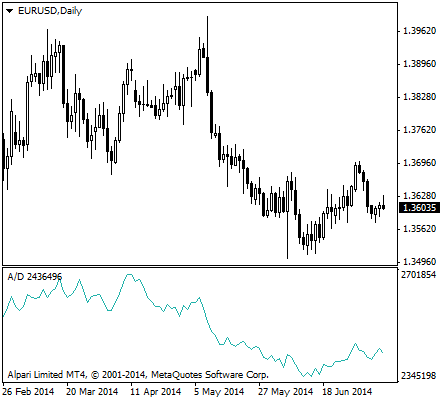In our other articles we talk about the importance of knowing the volume of a trade for a certain period of time. Relating it to a microeconomic example, consumers are more inclined to participate in a business where many transact with. Volume shows trust and strength but can also mean demise when high volume depicts preparation for a stock’s downfall. The OBV or On-Balance Volume is an indicator that shows the relationship of price and volume, and tells traders regarding the momentum of trades based on this relation.

Another technical indicator involving the property of volume in trades is the Accumulation Distribution Index. This indicator is used as a much more featureful version of the On-Balance Volume given the former’s complexity in calculation as compared to the much simpler OBV. There are some binary options brokers who prefer one from the other, but if the market sentiment is true, and all the trader needs to do is to look at points, then there should be no difference between the two indicators’ output.

The Accumulation Distribution Index, also known as the Accumulation Distribution Line or ADL, is a volume-based technical indicator that is intended to give the trader an idea of the cumulative money flow to and from a security. When traders know notice a positive or negative movement of money, that is the rate at which an asset’s price moves up or down, they know the right action to take and what options to purchase. Because the index is cummulative, historical price action matters very much in the current reading that traders monitor.

ADL was developed by Marc Chaikin, a stock analyst and CEO of Chaikin Stock Research, LLC. Because the indicator involved the summation of money flow, Chaikin originally called it the Cumulative Money Flow Line. An oscillator based on this indicator called the Chaikin oscillator is produced by subtracting a 10-day exponential moving average from a 3-day exponential moving average of the accumulation/distribution index. The Chaikin oscillator, being a meta-indicator, can give various call or put signals, all things being equal.

## Close Location Value (CLV)

In obtaining for the points that make up the Accumulation Distribution Line, first we need to have a multiplier that is calculated based on the relationship of the close price of an asset to its high-low range during the trading period. The Close Location Value or CLV gives us a picture of where a price closed relative to the high and low prices that occurred during the trade. Traders will be able to obtain signals from this value, and use them in other indicators that require for a point at which the price of an asset remains true.

The following is the calculation for CLV:

`CLV = (close — low) — (high — close) / (high — low)`

CLV is used to spot the tendency in the price movements. It is used as a measure in technical analysis to determine the “closest location” as a “value” where the price closed. The close location value is used in many indicators, but is most commonly known for its role in the calculation of the ADL. However, CLV can stand alone. For example, when the CLV and a stock price form a positive divergence, a bullish signal is considered, and vice versa. Also, when the CLV is crossing the zero line, a positive CLV is considered bullish, and vice versa.

The CLV ranges between +1 and -1, where a value of +1 means the close is equal to the high and a value of -1 means the close is equal to the day’s low. The implementing the Close Location Value into other technical indicators like the Accumulation Distribution Line is becoming more popular because it is often regarded as a better measure for the period’s activity than relying solely on the closing price. Furthermore, having a CLV as a determinant for other indicators gives an edge when it comes to bringing out the true market sentiment.

After calculating the CLV, the next step to obtaining the current ADL point is to multiply the CLV by the period’s volume and add the result to the previous point of the Accumulation Distribution Line. The second equation given below demonstrates formula. The CLV formula is restated to completely show the steps in obtaining the ADL. Again, the CLV ranges from -1 when the close is the low of the day, to +1 when it’s the high. For instance if the close is 3/4 the way up the high-low range for a certain period, then CLV is +0.5.

`CLV = (close — low) — (high — close) / (high — low)`

`ADL = ADLprev + Volume x CLV`

The ADL adds up volume multiplied by the CLV factor, that is, ADL = ADLprev + Volume x CLV. The starting point for the ADL, or the zero point, is arbitrary. Only the shape of the resulting indicator is used, and not the actual level of the total. Because the high, low, and close values change every trading period, a new CLV is constantly produced, showing significant changes in the ADL as well. It is these changes that binary options traders need to look out for in correctly interpreting this indicator.